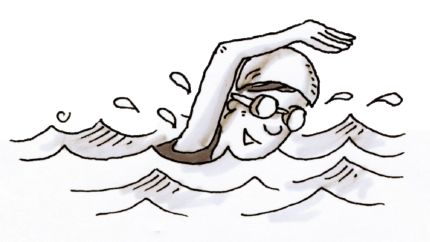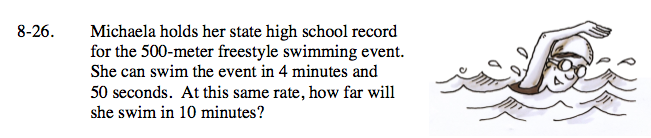Home > ACC7 > Chapter cc28 > Lesson cc28.1.2 > Problem8-26

8-26.Michaela holds her state high school record for the 500-meter freestyle swimming event. She can swim the event in 4 minutes and 50 seconds. At this same rate, how far will she swim in 10 minutes? Homework Help ✎Identify this as a proportional relationship problem.

You know that 500 meters is to 4 minutes and 50 seconds.
You will use this to find the distance that would go with 10 minutes.
Now use one of the three methods of solving proportions to find the answer to this problem.
Convert seconds to minutes to make the calculation easier (It will be a fraction).

$\text{Cross multiplication method: } \frac{500\text{ meters}}{4\frac{5}{6}\text{ minutes}}=\frac{\textit{x}}{10\text{ minutes}}$

$500(10)= \textit{x}\left(4\frac{5}{6}\right) \ \ \text{Solve for }x.$

1034.48 meters